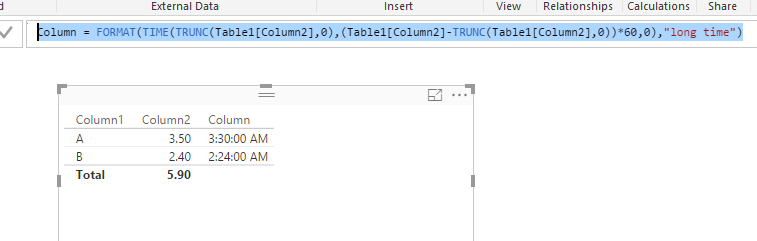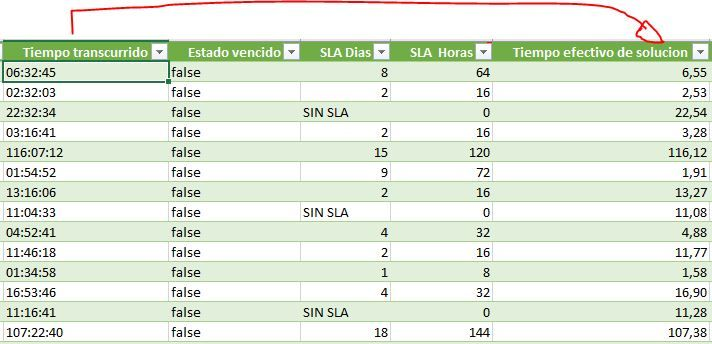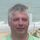cancel
Showing results for
Did you mean:Helper I

## Converting Decimal Hours to Time Format

Is there a nice easy way to convert decimal hours into hours/minutes in Power BI? A function that essentially does this?

For example, turning 3.5 hours into 3 hours and 30 minutes?

I've found a calculation to perform this function in Excel, but it apparently doesn't work for any values over 24.

10 REPLIES 10Anonymous
Not applicable

You might be able to use this DAX pattern, based on seconds rather than hours: https://community.powerbi.com/t5/Community-Blog/Aggregating-Duration-Time/ba-p/22486Microsoft

@sgross

In your scenario, you can take the integer part for Hours and use decimal part to calculate the Minutes. Populate both fields into TIME() function and format them into a time. Please refer to formula below:

`Column = FORMAT(TIME(TRUNC(Table1[Column2],0),(Table1[Column2]-TRUNC(Table1[Column2],0))*60,0),"long time")`Regards,New Member

Thank you for this, this worked really well.
Is there a way to stop it being a "text" format though as i would like to SUM the output of this.Anonymous
Not applicable

You can also do this in the Query editor:

= Time.Hour([Time])+(Time.Minute([Time)/60)+(Time.Minute([Time])/3600)

No problems with text format.Frequent Visitor

Hi

I have this case but in reverse. I need to convert a Time value to a Decimal value. In Excel I did not have any problems doing it because I took the "Tiempo Transcurrido (Time Lapsed)" field and multiplied it by 24.I tried some functions (Time, Value) but I can not find the result. I have tried how to extract each of these values and then convert them into a number but I can not find a function that does it.

If I try to convert that column to Time format, it gives me an errorFor me this value of Hour in decimal is very important for the calculations that I need to do.

I would greatly appreciate your help

Thank youFrequent Visitor

@jbolivar wrote:

Hi

I have this case but in reverse. I need to convert a Time value to a Decimal value. In Excel I did not have any problems doing it because I took the "Tiempo Transcurrido (Time Lapsed)" field and multiplied it by 24.I tried some functions (Time, Value) but I can not find the result. I have tried how to extract each of these values and then convert them into a number but I can not find a function that does it.

If I try to convert that column to Time format, it gives me an errorFor me this value of Hour in decimal is very important for the calculations that I need to do.

I would greatly appreciate your help

Thank you

Friends,

I have solved this case using Power Query M function

=Number.FromText(Text.BeforeDelimiter([Tiempo transcurrido],":",0)) + (Number.FromText(Text.BetweenDelimiters([Tiempo transcurrido],":",":")))/60 + (Number.FromText(Text.AfterDelimiter([Tiempo transcurrido],":",1)))/3600.

Thank youCommunity Champion

An alternative would be:

```= List.Sum(
List.Transform(
List.Zip({
Text.Split(
[Tiempo transcurrido],
":"),
{1,60,3600}}),
each Number.From(_{0})/_{1})
)```
Specializing in Power Query Formula Language (M)Frequent Visitor

@MarcelBeug wrote:

An alternative would be:

```= List.Sum(
List.Transform(
List.Zip({
Text.Split(
[Tiempo transcurrido],
":"),
{1,60,3600}}),
each Number.From(_{0})/_{1})
)```

Excellent..it worked too

Thank youHelper I

Hello @v-sihou-msft, thanks for your help. I'm completely new to this, so I could use a little more direction. Are you entering those functions using R Script?

Is there any way to achieve the same effect in the Query Editor? Creating a seperate column with the converted values?Community Champion

If you want to exceed 24 hours, then you need Duration format, like:

Duration.From([DecimalTime]/24)

Specializing in Power Query Formula Language (M)Announcements#### Exclusive opportunity for Women!

Join us for a free, hands-on Microsoft workshop led by women trainers for women where you will learn how to build a Dashboard in a Day!#### Power Platform Conference-Power BI and Fabric Sessions

Join us Oct 1 - 6 in Las Vegas for the Microsoft Power Platform Conference.Top Solution Authors
Top Kudoed Authors
Users online (2,756)